Superconductivity

Start this free course now. Just create an account and sign in. Enrol and complete the course for a free statement of participation or digital badge if available.

Free course

# 2.4 Critical magnetic field

An important characteristic of a superconductor is that its normal resistance is restored if a sufficiently large magnetic field is applied. The nature of this transition to the normal state depends on the shape of the superconductor and the orientation of the magnetic field, and it is also different for pure elements and for alloys. In this subsection we describe the behaviour in the simplest situation; we shall discuss other more complex behaviour in Section 4.

If an increasing magnetic field is applied parallel to a long thin cylinder of tin at a constant temperature below the critical temperature, then the cylinder will make a transition from the superconducting state to the normal state when the field reaches a well-defined strength. This field at which the superconductivity is destroyed is known as the critical magnetic field strength, B﻿c. If the field is reduced, with the temperature held constant, the tin cylinder returns to the superconducting state at the same critical field strength B﻿c.

Experiments indicate that the critical magnetic field strength depends on temperature, and the form of this temperature dependence is shown in Figure 11 for several elements. At very low temperatures, the critical field strength is essentially independent of temperature, but as the temperature increases, the critical field strength drops, and becomes zero at the critical temperature. At temperatures just below the critical temperature it requires only a very small magnetic field to destroy the superconductivity.

The temperature dependence of the critical field strength is approximately parabolic: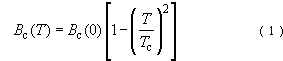where Bc(0) is the extrapolated value of the critical field strength at absolute zero and T﻿c is the critical temperature. The curves in Figure 11 indicate that a superconductor with a high critical temperature T﻿c has a high critical field strength B﻿c at T = 0 K, and Table 1 confirms this correlation for a larger number of superconducting elements.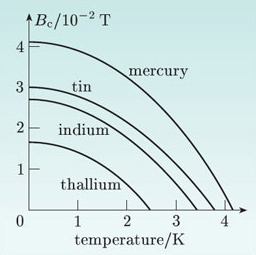Figure 11 The temperature dependences of the critical magnetic field strengths of mercury, tin, indium and thallium.

## Table 1 The critical temperatures Tc and critical magnetic field strengths Bc(0) for various superconducting elements.

T﻿c﻿/﻿KB﻿c﻿(﻿0﻿)﻿/﻿mT
aluminium1.210
indium3.428
mercury4.241
tantalum4.583
thallium2.418
tin3.731
titanium0.405.6
zinc0.855.4

## Exercise 2

Estimate the magnetic field strength necessary to destroy superconductivity in a sample of lead at 4.2 K.

From Table 1, for lead T﻿c = 7.2 K and B﻿c﻿(﻿0﻿)  =  0.080 T. From Equation 1,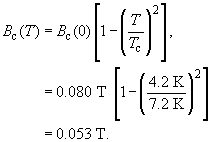It is interesting to compare the magnetic behaviour of a superconducting element with typical curves for diamagnetic, paramagnetic and ferromagnetic materials. The magnetic behaviour of magnetic materials can be represented by B versus H graphs. Figure 12a shows the behaviour of typical diamagnetic and paramagnetic materials. Note that we have plotted μ0H on the horizontal axis rather than H, so that both axes use the same unit (tesla). The straight lines plotted correspond to the relationship B = μ﻿μ﻿0﻿H, with μ slightly smaller than unity for the diamagnetic material and slightly greater than unity for the paramagnetic material. The behaviour of a ferromagnet, shown in Figure 12b, is quite different, with B ≫ μ﻿0﻿H, and a highly non-linear and irreversible curve until the magnetisation saturates, after which B increases linearly with H.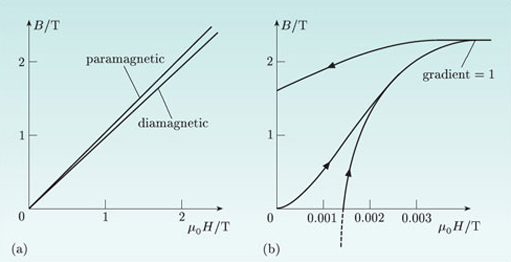Figure 12 Graphs showing B versus μ﻿0﻿H for (a) diamagnetic and paramagnetic materials, and (b) a ferromagnetic material. Note the different scales for the μ﻿0﻿H-axes in the two graphs.

Compare these graphs with Figure 13, which shows the B﻿-﻿H curve for a superconducting cylinder of tin, with the field parallel to its axis. The field strength B within the superconductor is zero when μ0﻿H is less than the critical field strength B﻿c; the superconductor behaves like a perfect diamagnetic material and completely excludes the field from its interior. But then B jumps abruptly to a value B﻿c, and at higher fields the tin cylinder obeys the relationship B﻿μ0﻿H, since the material is weakly diamagnetic in its normal state, with μ = 0.9998. The linear graphs in Figure 12a are similar to those for a superconductor above the critical field strength.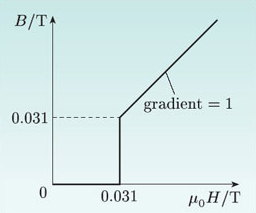Figure 13 Graph showing B versus μ﻿0﻿H for a tin cylinder, aligned parallel to the field.

SMT359_1